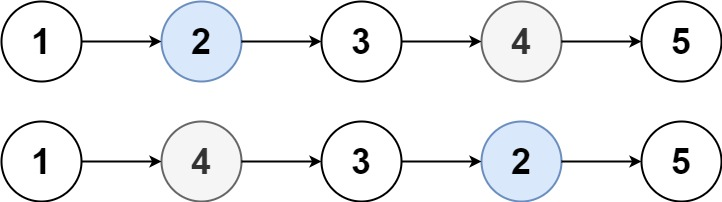# GeetCode Hub

You are given the `head` of a linked list, and an integer `k`.

Return the head of the linked list after swapping the values of the `kth` node from the beginning and the `kth` node from the end (the list is 1-indexed).

Example 1:```Input: head = [1,2,3,4,5], k = 2
Output: [1,4,3,2,5]
```

Example 2:

```Input: head = [7,9,6,6,7,8,3,0,9,5], k = 5
Output: [7,9,6,6,8,7,3,0,9,5]
```

Example 3:

```Input: head = , k = 1
Output: 
```

Example 4:

```Input: head = [1,2], k = 1
Output: [2,1]
```

Example 5:

```Input: head = [1,2,3], k = 2
Output: [1,2,3]
```

Constraints:

• The number of nodes in the list is `n`.
• `1 <= k <= n <= 105`
• `0 <= Node.val <= 100`

/** * Definition for singly-linked list. * public class ListNode { * int val; * ListNode next; * ListNode() {} * ListNode(int val) { this.val = val; } * ListNode(int val, ListNode next) { this.val = val; this.next = next; } * } */ class Solution { public ListNode swapNodes(ListNode head, int k) { } }